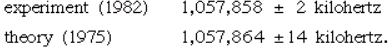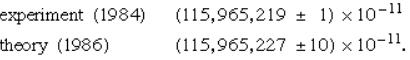# Quantum Electrodynamics Physics Notes | EduRev

## Physics : Quantum Electrodynamics Physics Notes | EduRev

The document Quantum Electrodynamics Physics Notes | EduRev is a part of the Physics Course Modern Physics for IIT JAM.
All you need of Physics at this link: Physics

The application of quantum theory to the interaction between electrons and radiation requires a quantum treatment of Maxwell’s field equations, which are the foundations of electromagnetism, and the relativistic theory of the electron formulated by Dirac (see above Electron spin and antiparticles). The resulting quantum field theory is known as quantum electrodynamics, or QED.
QED accounts for the behaviour and interactions of electrons, positrons, and photons. It deals with processes involving the creation of material particles from electromagnetic energy and with the converse processes in which a material particle and its antiparticle annihilate each other and produce energy. Initially the theory was beset with formidable mathematical difficulties, because the calculated values of quantities such as the charge and mass of the electron proved to be infinite. However, an ingenious set of techniques developed (in the late 1940s) by Hans Bethe, Julian S. Schwinger, Tomonaga Shin’ichirō, Richard P. Feynman, and others dealt systematically with the infinities to obtain finite values of the physical quantities. Their method is known as renormalization. The theory has provided some remarkably accurate predictions.
According to the Dirac theory, two particular states in hydrogen with different quantum numbers have the same energy. QED, however, predicts a small difference in their energies; the difference may be determined by measuring the frequency of the electromagnetic radiation that produces transitions between the two states. This effect was first measured by Willis E. Lamb, Jr., and Robert Retherford in 1947. Its physical origin lies in the interaction of the electron with the random fluctuations in the surrounding electromagnetic field. These fluctuations, which exist even in the absence of an applied field, are a quantum phenomenon. The accuracy of experiment and theory in this area may be gauged by two recent values for the separation of the two states, expressed in terms of the frequency of the radiation that produces the transitions:An even more spectacular example of the success of QED is provided by the value for μe, the magnetic dipole moment of the free electron. Because the electron is spinning and has electric charge, it behaves like a tiny magnet, the strength of which is expressed by the value of μe. According to the Dirac theory, μe is exactly equal to μB = eℏ/2me, a quantity known as the Bohr magneton; however, QED predicts that μe = (1 + a)μB, where a is a small number, approximately 1/860. Again, the physical origin of the QED correction is the interaction of the electron with random oscillations in the surrounding electromagnetic field. The best experimental determination of μe involves measuring not the quantity itself but the small correction term μe − μB. This greatly enhances the sensitivity of the experiment. The most recent results for the value of a areSince a itself represents a small correction term, the magnetic dipole moment of the electron is measured with an accuracy of about one part in 1011. One of the most precisely determined quantities in physics, the magnetic dipole moment of the electron can be calculated correctly from quantum theory to within about one part in 1010.

Offer running on EduRev: Apply code STAYHOME200 to get INR 200 off on our premium plan EduRev Infinity!

## Modern Physics for IIT JAM

20 videos|44 docs|15 tests

,

,

,

,

,

,

,

,

,

,

,

,

,

,

,

,

,

,

,

,

,

;